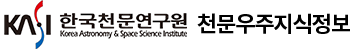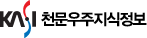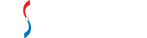#통합검색

#### 통합검색

인기검색어### 한국천문학회지

• 홈으로 이동1968년 ~ 2019년까지 1,094 건한국천문학회지를 격월간 확인하실 수 있습니다.

• The Korean Astronomical Society (The Korean Astronomical Society)
• 계간 (Quarterly)
• ISSN : 1225-4614 (ISSN : 1225-4614)
• DB구축현황 : 1,094건 (DB Construction : 1,094 Articles)
안내사항
총 게시글 1,094 페이지 3/110
21
• Pak, Sungmin
• Journal of the Korean astronomical society = 천문학회지
• 51, n.4
• pp.111-117
• 2018
• 원문 바로보기
A numerical method is proposed to calculate the response of detectors measuring particle energies from incident isotropic fluxes of electrons and positive ions. The isotropic flux is generated by injecting particles moving radially inward on a hypothetical, spherical surface encompassing the detectors. A geometric projection of the field-of-view from the detectors onto the spherical surface allows for the identification of initial positions and momenta corresponding to the clear field-of-view of the detectors. The contamination of detector responses by particles penetrating through, or scattering off, the structure is also similarly identified by tracing the initial positions and momenta of the detected particles. The relative contribution from the contaminating particles is calculated using GEANT4 to obtain the geometric factor of the instrument as a function of the energy. This calculation clearly shows that the geometric factor is a strong function of incident particle energies. The current investigation provides a simple and decisive method to analyze the instrument geometric factor, which is a complicated function of contributions from the anticipated field-of-view particles, together with penetrating or scattered particles.
22
• Zheng, Yanfang
• Journal of the Korean astronomical society = 천문학회지
• 51, n.3
• pp.65-71
• 2018
• 원문 바로보기
The near real-time speckle masking reconstruction technique has been developed to accelerate the processing of solar images to achieve high resolutions for ground-based solar telescopes. However, the reconstruction of solar subimages in such a speckle reconstruction is very time-consuming. We design and implement a new parallel speckle masking reconstruction algorithm based on the Compute Unified Device Architecture (CUDA) on General Purpose Graphics Processing Units (GPGPU). Tests are performed to validate the correctness of our program on NVIDIA GPGPU. Details of several parallel reconstruction steps are presented, and the parallel implementation between various modules shows a significant speed increase compared to the previous serial implementations. In addition, we present a comparison of runtimes across serial programs, the OpenMP-based method, and the new parallel method. The new parallel method shows a clear advantage for large scale data processing, and a speedup of around 9 to 10 is achieved in reconstructing one solar subimage of $256{\times}256pixels$ . The speedup performance of the new parallel method exceeds that of OpenMP-based method overall. We conclude that the new parallel method would be of value, and contribute to real-time reconstruction of an entire solar image.
23
• Seo, Hyunjong
• Journal of the Korean astronomical society = 천문학회지
• 51, n.3
• pp.49-63
• 2018
• 원문 바로보기
We carry out a study of Sub-Millimeter Galaxies (SMGs) in the AKARI NEP-Deep field using the James Clerk Maxwell Telescope (JCMT) SCUBA-2 $850{\mu}m$ source catalog, released as part of the SCUBA-2 Cosmology Legacy Survey (S2CLS) program. The SCUBA-2 $850{\mu}m$ map has a root mean square (rms) noise of $1.2mJy\;beam^{-1}$ and covers an area of $0.60degree^2$ . We find four SMGs which have counterparts to Herschel sources with spectroscopic redshifts in the literature. In addition, three dust obscured galaxies (DOGs) detected in Herschel bands are selected as a comparison sample. We derive IR luminosities of SMGs using the CIGALE code, which are similar to those of high redshift SMGs from previous studies. The contribution of AGN to the total IR luminosity in SMGs (2%-11%) is smaller than the lower limit for the one in DOGs (19%-35%), which is consistent with the expectation from the evolutionary scenario of massive galaxies. We search for SMGs in overdense regions as protocluster candidates and investigate four regions, including candidates around three DOGs. Finally, we argue that follow-up spectroscopic observation for the NEP-Deep field will provide crucial information to understand the role of SMGs in the evolution of massive galaxies
24
• Li, Lin-Sen
• Journal of the Korean astronomical society = 천문학회지
• 51, n.1
• pp.1-4
• 2018
• 원문 바로보기
We study the pseudo-synchronous orbital motion of a binary system on the main sequence. The equations of the pseudo-synchronous orbit are derived up to $O(e^4)$ where e is the eccentricy of the orbit. We integrate the equations to present their solutions. The theoretical results are applied to the evolution of the orbit and spin of the binary star Y Cygni, which has a current eccentricity of $e_0\;=\;0.142$ . We tabulate our numerical results for the evolution of the orbit and spin per century. The numerical results for the semi-major axes and rotational angular velocities in the evolutional time scales of three stages (synchronization, circularization, and collapse time scale) are also tabulated. Synchronization is achieved in about $5{\times}10^3\;years$ followed by circularization lasting about $1{\times}10^5\;years$ before decaying in $2{\times}10^5\;years$ .
25
• Seo, Jeongbhin
• Journal of the Korean astronomical society = 천문학회지
• 51, n.2
• pp.37-48
• 2018
• 원문 바로보기
Massive stars blow powerful stellar winds throughout their evolutionary stages from the main sequence to Wolf-Rayet phases. The amount of mechanical energy deposited in the interstellar medium by the wind from a massive star can be comparable to the explosion energy of a core-collapse supernova that detonates at the end of its life. In this study, we estimate the kinetic energy deposition by massive stars in our Galaxy by considering the integrated Galactic initial mass function and modeling the stellar wind luminosity. The mass loss rate and terminal velocity of stellar winds during the main sequence, red supergiant, and Wolf-Rayet stages are estimated by adopting theoretical calculations and observational data published in the literature. We find that the total stellar wind luminosity due to all massive stars in the Galaxy is about ${\mathcal{L}}_w{\approx}1.1{\times}10^{41}erg\;s^{-1}$ , which is about 1/4 of the power of supernova explosions, ${\mathcal{L}}_{SN}{\approx}4.8{\times}10^{41}erg\;s^{-1}$ . If we assume that ~ 1 - 10 % of the wind luminosity could be converted to Galactic cosmic rays (GCRs) through collisonless shocks such as termination shocks in stellar bubbles and superbubbles, colliding-wind shocks in binaries, and bow-shocks of massive runaway stars, stellar winds might be expected to make a significant contribution to GCR production, though lower than that of supernova remnants.
26
• Yang, Heesu
• Journal of the Korean astronomical society = 천문학회지
• 51, n.2
• pp.27-36
• 2018
• 원문 바로보기
In a solar coronagraph, the most important component is an occulter to block the direct light from the disk of the sun. Because the intensity of the solar outer corona is $10^{-6}$ to $10^{-10}$ times of that of the solar disk ( $I_{\odot}$ ), it is necessary to minimize scattering at the optical elements and diffraction at the occulter. Using a Fourier optic simulation and a stray light test, we investigated the performance of a compact coronagraph that uses an external truncated-cone occulter without an internal occulter and Lyot stop. In the simulation, the diffracted light was minimized to the order of $7.6{\times}10^{-10}I_{\odot}$ when the cone angle ${\theta}_c$ was about $0.39^{\circ}$ . The performance of the cone occulter was then tested by experiment. The level of the diffracted light reached the order of $6{\times}10^{-9}I_{\odot}$ at ${\theta}_c=0.40^{\circ}$ . This is sufficient to observe the outer corona without additional optical elements such as a Lyot stop or inner occulter. We also found the manufacturing tolerance of the cone angle to be $0.05^{\circ}$ , the lateral alignment tolerance was $45{\mu}m$ , and the angular alignment tolerance was $0.043^{\circ}$ . Our results suggest that the physical size of coronagraphs can be shortened significantly by using a cone occulter.
27
• Chang, Seok-Jun
• Journal of the Korean astronomical society = 천문학회지
• 51, n.1
• pp.5-16
• 2018
• 원문 바로보기
We investigate the escape of $Ly{\beta}$ from emission nebulae with a significant population of excited hydrogen atoms in the level n = 2, rendering them optically thick in $H{\alpha}$ . The transfer of $Ly{\beta}$ line photons in these optically thick regions is complicated by the presence of another scattering channel leading to re-emission of $H{\alpha}$ , alternating their identities between $Ly{\beta}$ and $H{\alpha}$ . In this work, we develop a Monte Carlo code to simulate the transfer of $Ly{\beta}$ line photons incorporating the scattering channel into $H{\alpha}$ . Both $H{\alpha}$ and $Ly{\beta}$ lines are formed through diffusion in frequency space, where a line photon enters the wing regime after a fairly large number of resonance scatterings with hydrogen atoms. Various line profiles of $H{\alpha}$ and $Ly{\beta}$ emergent from our model nebulae are presented. It is argued that the electron temperature is a critical parameter which controls the flux ratio of emergent $Ly{\beta}$ and $H{\alpha}$ . Specifically for $T\;=\;3{\times}10^4\;K$ and $H{\alpha}$ line center optical depth $\tau{\alpha}\;=\;10$ , the number flux ratio of emergent $Ly{\beta}$ and $H{\alpha}$ is ~ 49 percent, which is quite significant. We propose that the leaking $Ly{\beta}$ can be an interesting source for the formation of $H{\alpha}$ wings observed in many symbiotic stars and active galactic nuclei. Similar broad $H{\alpha}$ wings are also expected in $Ly{\alpha}$ emitting halos found in the early universe, which can be potentially probed by the James Webb Telescope in the future.
28
• Bang, Tae-Yang
• Journal of the Korean astronomical society = 천문학회지
• 51, n.1
• pp.17-25
• 2018
• 원문 바로보기
Detecting exoplanets around giant stars sheds light on the later-stage evolution of planetary systems. We observed the M giant HD 18438 and the K giant HD 158996 as part of a Search for Exoplanets around Northern circumpolar Stars (SENS) and obtained 38 and 24 spectra from 2010 to 2017 using the high-resolution Bohyunsan Observatory Echelle Spectrograph (BOES) at the 1.8m telescope of Bohyunsan Optical Astronomy Observatory in Korea. We obtained precise RV measurements from the spectra and found long-period radial velocity (RV) variations with period 719.0 days for HD 18438 and 820.2 days for HD 158996. We checked the chromospheric activities using Ca $\text\tiny{II}$ H and $H{\alpha}$ lines, HIPPARCOS photometry and line bisectors to identify the origin of the observed RV variations. In the case of HD 18438, we conclude that the observed RV variations with period 719.0 days are likely to be caused by the pulsations because the periods of HIPPARCOS photometric and $H{\alpha}$ EW variations for HD 18438 are similar to that of RV variations in Lomb-Scargle periodogram, and there are no correlations between bisectors and RV measurements. In the case of HD 158996, on the other hand, we did not find any similarity in the respective periodograms nor any correlation between RV variations and line bisector variations. In addition, the probability that the real rotational period can be as longer than the RV period for HD 158996 is only about 4.3%. Thus we conclude that observed RV variations with a period of 820.2 days of HD 158996 are caused by a planetary companion, which has the minimum mass of 14.0 $M_{Jup}$ , the semi-major axis of 2.1 AU, and eccentricity of 0.13 assuming the stellar mass of $1.8 M_{\odot}$ . HD 158996 is so far one of the brightest and largest stars to harbor an exoplanet candidate.
29
• Magara, Tetsuya
• Journal of the Korean astronomical society = 천문학회지
• 50, n.6
• pp.179-184
• 2017
• 원문 바로보기
We present a new method for solving an inverse problem of flux emergence which transports subsurface magnetic flux from an inaccessible interior to the surface where magnetic structures may be observed to form, such as solar active regions. To make a quantitative evaluation of magnetic structures having various characteristics, we derive physical properties of subsurface magnetic field that characterize those structures formed through flux emergence. The derivation is performed by inversion from an evolutionary relation between two observables obtained at the surface, emerged magnetic flux and injected magnetic helicity, the former of which provides scale information while the latter represents the configuration of magnetic field.
30
• Lee, Ki-Won
• Journal of the Korean astronomical society = 천문학회지
• 50, n.6
• pp.191-200
• 2017
• 원문 바로보기
We analyze the time data recorded in Korean astronomical almanacs for the years from 1913 to 1945, which belong to the period in which Japan occupied Korea (1910-1945). These almanacs, published by Japanese scholars, differ from previous almanacs in terms of organization, content, and calendrical methods. In this study, we first extract twelve kinds of time data from the almanacs at the following times: solar terms, rising and setting of the Sun and Moon, transit of the Sun, phases of the Moon (i.e., new Moon, first quarter Moon, full Moon, and last quarter Moon), and eclipses of the Sun and Moon. Then, we compare the time data with that obtained from modern calculations. Even though all time data in the almanacs are tabulated in units of minutes, we calculate the data in units of seconds and determine the root mean square (RMS) deviation values for each kind of time data to estimate the accuracy of the data. Our findings are as follows: First, the kind and tabulation method of time data changes several times. For instance, solar transit time is listed only for six years from 1937 to 1942. Second, the times of two equinoxes and those of a new Moon are considerably close to midnight. Third, there are some typographical errors in the almanacs, particularly in the times of moonrise and moonset. Fourth, the contact times for lunar eclipses represent the times of the umbra and not of the penumbra, which is different from the times for solar eclipses. Finally, the RMS deviation values are approximately 0.5 min on average in all kinds of time data, even though they show slightly large differences in the times related to the Moon. In conclusion, we believe that this study is useful for investigating the time data in the almanacs of other East Asian countries that were published during the same period, such as China, Japan, and Manchuria.